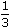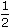# Aptitude - Pipes and Cistern

Exercise : Pipes and Cistern - General Questions
1.
Three pipes A, B and C can fill a tank from empty to full in 30 minutes, 20 minutes, and 10 minutes respectively. When the tank is empty, all the three pipes are opened. A, B and C discharge chemical solutions P,Q and R respectively. What is the proportion of the solution R in the liquid in the tank after 3 minutes?
 5 11
 6 11
 7 11
 8 11
Explanation:

 Part filled by (A + B + C) in 3 minutes = 31 + 1 + 1=3 x 11= 11 . 30 20 10 60 20

 Part filled by C in 3 minutes = 3 . 10Required ratio =3 x 20= 6 . 10 11 11

2.
Pipes A and B can fill a tank in 5 and 6 hours respectively. Pipe C can empty it in 12 hours. If all the three pipes are opened together, then the tank will be filled in:
 1 13 hours 17
 2 8 hours 11
 3 9 hours 17
 4 1 hours 2
Explanation:

 Net part filled in 1 hour1 + 1 - 1= 17 . 5 6 12 60The tank will be full in 60 hours i.e., 3 9 hours. 17 17

3.
A pump can fill a tank with water in 2 hours. Because of a leak, it took 2hours to fill the tank. The leak can drain all the water of the tank in:
 4 1 hours 3
7 hours
8 hours
14 hours
Explanation:

 Work done by the leak in 1 hour =1 - 3= 1 . 2 7 14Leak will empty the tank in 14 hrs.

4.
Two pipes A and B can fill a cistern in 37minutes and 45 minutes respectively. Both pipes are opened. The cistern will be filled in just half an hour, if the B is turned off after:
5 min.
9 min.
10 min.
15 min.
Explanation:

Let B be turned off after x minutes. Then,

Part filled by (A + B) in x min. + Part filled by A in (30 -x) min. = 1.x2 + 1+ (30 - x). 2 = 1 75 45 7511x + (60 -2x) = 1 225 7511x + 180 - 6x = 225.x = 9.

5.
A tank is filled by three pipes with uniform flow. The first two pipes operating simultaneously fill the tank in the same time during which the tank is filled by the third pipe alone. The second pipe fills the tank 5 hours faster than the first pipe and 4 hours slower than the third pipe. The time required by the first pipe is:
6 hours
10 hours
15 hours
30 hours
Explanation:

Suppose, first pipe alone takes x hours to fill the tank .

Then, second and third pipes will take (x -5) and (x - 9) hours respectively to fill the tank.1 + 1 = 1 x (x - 5) (x - 9)x - 5 + x = 1 x(x - 5) (x - 9)(2x - 5)(x - 9) = x(x - 5)x2 - 18x + 45 = 0

(x - 15)(x - 3) = 0x = 15.    [neglecting x = 3]